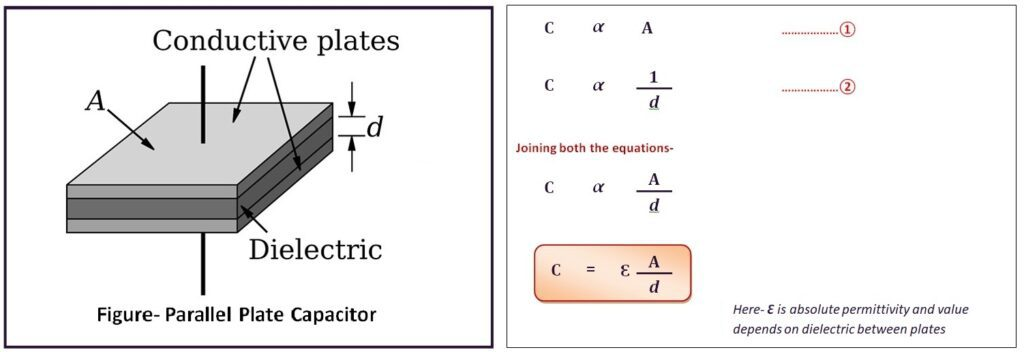# Capacitive Transducer

Capacitive transducer is also called as Variable capacitance transducer. When a physical quantity such as temperature, pressure, displacement, vibration, force etc. make changes in capacitance or relative capacitance for the sensor, these sensors are termed as capacitive sensor or variable capacitance transducers.

## Working principle of capacitive transducer-

Since we know that capacitance (C) (Measured in Farad, µF, pF) of a parallel plate capacitor is proportional to the effective area of plate (A) and inversely proportional to the distance (d) between both the plates i.e.-### What is Dielectric and Dielectric Constant?

Dielectric substance is defined as a material which has poor electrical conductivity but it has ability to store electrical charge (due to dielectric polarization).

And Dielectric constant (ɛr) of a substance is defined as the ratio of the permittivity of the substance (ɛ) to the permittivity of the free space (ɛ0). This is also termed as relative permittivity. Basically dielectric constant shows capability of storing electric charge by the substance with respect to capability of storing charge by free space.From the formula, it is understood that the variable capacitance transducer can be varied by the following parameters–

• The effective area (A) of the plates of these transducers can vary its capacitance value.
• Distance (d) between the plates can vary the transducers’ capacitance value.
• Change in dielectric constant (ɛr) can also vary the capacitance of this transducer.

These three methods are applied in variable capacitance transducers. The various configurations of capacitive transducers are as follows-

• Parallel plate capacitance with rectangular plate configuration.
• Cylindrical capacitor transducer configuration.
• Semi circular parallel plates configuration.
• Change in dielectric between parallel plates configuration.As conclusion, if a process variable makes change in any of three parameters for capacitive transducer, will change in capacitance of the same, which can be detected and measured by bridge circuits by detecting change in voltage across it or change in charge stored or current flowing through it and can be calibrated in unit of measurement of process variable.

## Application of capacitive transducer-

Some useful applications of capacitive transducers base on above mentioned principles are shown below in table-

 Level Measurement Level for conducting liquid/ fluid based on change in distance between parallel plate capacitor Level Measurement Level for Non-conducting liquid/ fluid based on change in dielectric between parallel plate capacitor Thickness measurement Used for thickness measurement of dielectric material with the help of using change in distance between plates principle Linear Displacement measurement Displacement measurement either by changing plate distance, or change in effective cross section area between plate/ cylindrical core, or by causing movement of dielectric material core between plate Force measurement By converting force into displacement using primary transducer and then Displacement measurement either by changing plate distance, or change in effective cross section area between plate, or by causing movement of dielectric material core between plate Pressure measurement Used as diaphragm capsule with a fixed plate and a pressure sensing diaphragm as movable plate filled with dielectric such as silicon or glycerin fluid. Based on change in distance between plates. Or by converting pressure into displacement using primary transducers and measure displacement. Angular displacement/ rotary motion Measurement Angular displacement/ rotary motion Measurement by changing effecting area between plates of semi circular capacitor. Torque measurement By converting force into rotary motion using primary transducer and perform Angular displacement/ rotary motion Measurement by changing effecting area between plates of semi circular capacitor.

Related Search:-